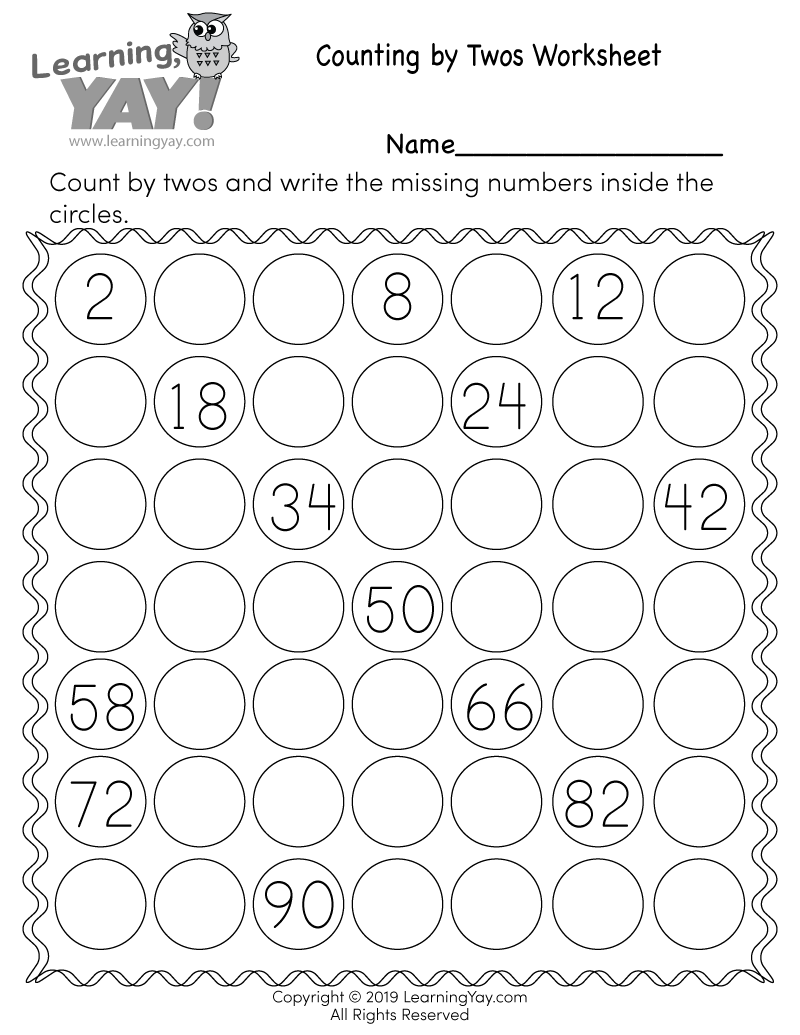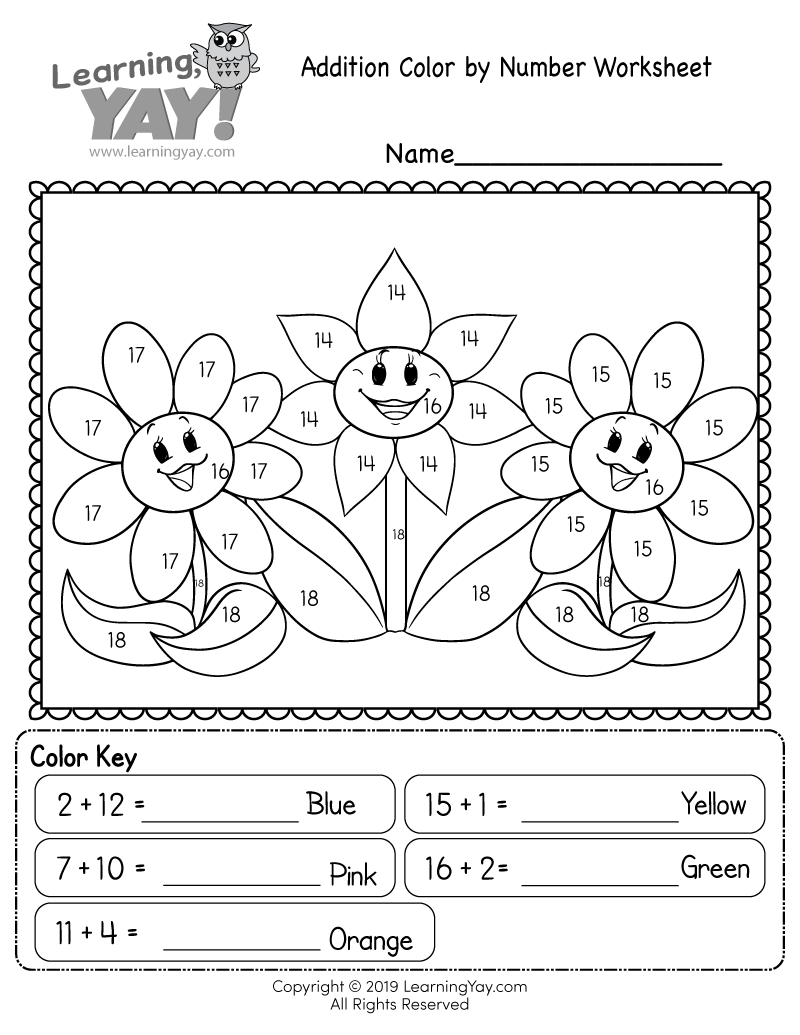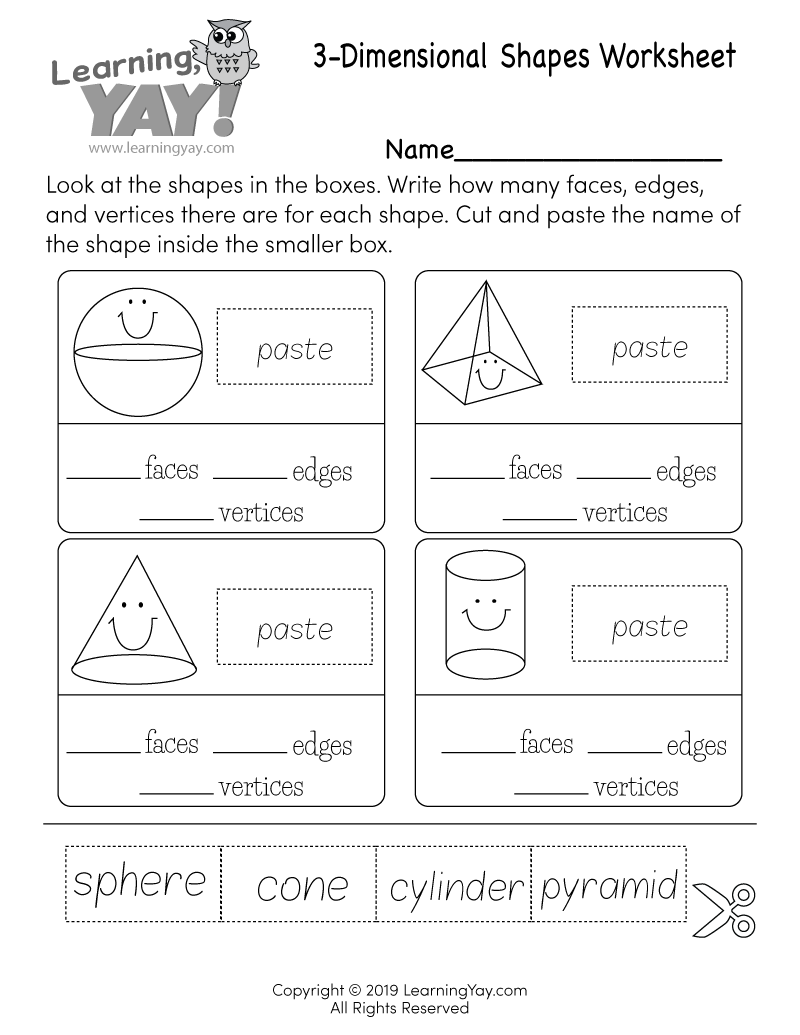# Skip Counting by 2s Worksheet

First graders can practice counting by 2s to 100 and write in all the missing numbers along the way with this free math worksheet. Skip counting is a great way for children to learn how addition works.This free printable math worksheet is aligned to first grade Common Core standards. It is an excellent resource for teachers and parents who are teaching the following standard.
##### 1.NBT.A.1 - Common Core ID

Count to 120, starting at any number less than 120. In this range, read and write numerals and represent a number of objects with a written numeral. Understand place value.

Common Core » 1st Grade Math Standards » Number & Operations in Base Ten » Extend the counting sequence. » 1.NBT.A.1

## There are multiple ways to get this worksheet.

### You might also like these math worksheets: